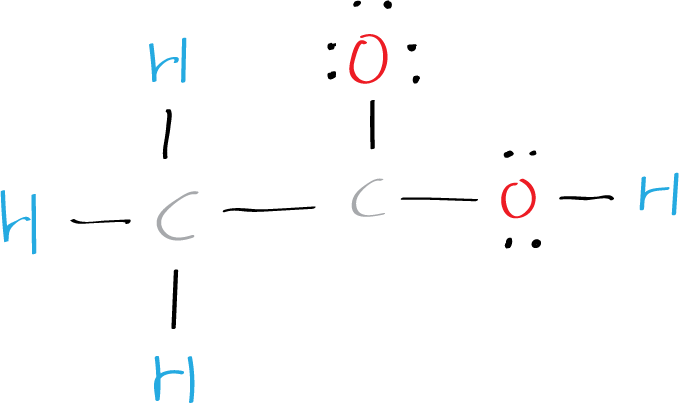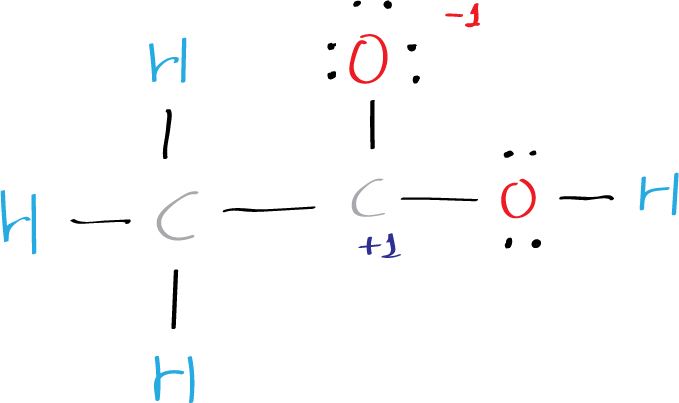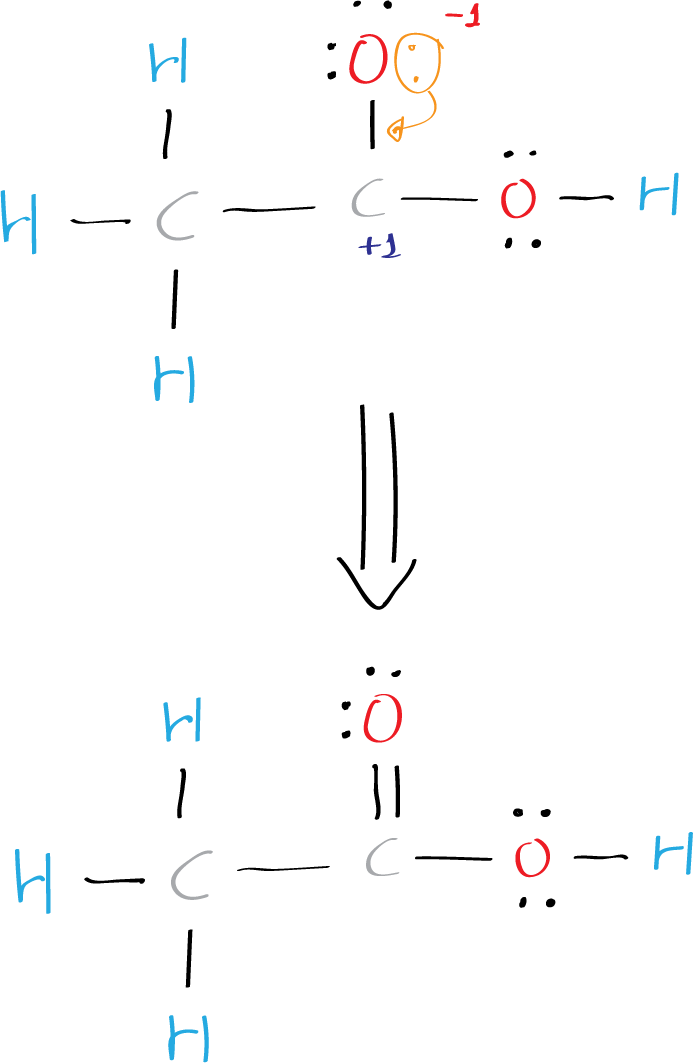# Lewis structure of acetic acid CH3COOH (etanoic acid)

## What is the Lewis structure of acetic acid?

The Lewis structure of acetic acid (CH3COOH) consists of two carbon (C) atoms double-bonded to one oxygen (O) atom and a single bond to another oxygen atom. Each carbon atom is also bonded to three hydrogen (H) atoms, and the remaining oxygen atom has two lone pairs of electrons.

To draw the Lewis structure of acetic acid CH3COOH, follow these step-by-step instructions.

## Step 1: Count the total number of valence electrons

Valence electrons are the outermost electrons in an atom that are involved in chemical bonding. To determine the number of valence electrons in hydrogen sulfide, add the number of valence electrons in each atom. Hydrogen has one valence electron, while oxygen has six valence electrons, and carbon has four electrons. Therefore, CH3COOH has a total of twenty four valence electrons.

4×(1) + 2×(6) + 2×(4) = 24

## Step 2: Determine the central atom

In CH3COOH, there is no a central atom. The organic molecule is formed by a carbon chain and oxygen are attached to one carbon. The remaining oxygen atoms are bonded 3 to one carbon and one to a oxygen atom. Hydrogen can only form one bond, while carbon can form four bonds, and oxygen up to two bonds.

## Step 3: Connect the atoms

Draw the carbon chain, then put the oxygens and finally the hydrogens. Connect 3 hydrogen atoms to the carbon atom with a single bond and another to one oxygen.

## Step 4: Place the remaining electrons

Place the remaining six valence electrons around the oxygen atoms in pairs. One oxygen will have two lone pairs and two bonding pairs of electrons in the atom attached to hydrogen. The remaining oxygen bonded to carbon will have three lone pairs and one bonding pair of electrons. Lone pairs are non-bonding pairs of electrons.Lewis structure of acetic acid CH3COOH (ethanoic acid) (step 4 place the remaining electrons on the atoms)

## Step 5: Check the octet rule (formal charges)

In the Lewis structure of acetic acid CH3COOH (ethanoic acid), oxygen has 8 electrons around it, one carbon 8 electrons, and the other oxygen just 6 electrons (+1 charge). Each hydrogen atom has two electrons around it.

The formal charge is a measure of the distribution of electrons in a molecule. It is calculated by subtracting the number of non-bonding electrons and half of the bonding electrons from the number of valence electrons in an atom. The formal charge of an atom should be as close to zero as possible. To check the formal charges in water, we use the following formula:

Formal charge = Valence electrons – Non-bonding electrons – 1/2 (Bonding electrons)

For the oxygen atom attached to hydrogen, the formal charge is:

6 – 4 – 1/2(4) = 0

For the oxygen atom attached to carbon only, the formal charge is:

6 – 6 – 1/2(2) = -1

For the carbon atom attached to 3 hydrogens and one carbon, the formal charge is:

4 – 0 – 1/2(8) = 0

For the carbon atom attached to 2 oxygens and one carbon, the formal charge is:

4 – 0 – 1/2(6) = +1

For each hydrogen atom, the formal charge is:

1 – 0 – 1/2(2) = 0

This does not satisfies the octet rule, which states that atoms tend to bond in such a way that each atom has eight valence electrons around it, so, charges +1 and -1 has to be added.Lewis structure of acetic acid CH3COOH (ethanoic acid) step 5: Check the octet rule (formal charges)

Two bonded atoms (oxygen and carbon) has +1 and -1 charge and in total is 0. Therefore, to simplify the structure, one must move an electron pair from oxygen to form a double C=O bond. Thus, the final structure can be easily drawn without partial charges on the C and O atoms.Lewis structure of acetic acid CH3COOH (ethanoic acid) step 5: Check the octet rule (formal charges)

## Step 6: Draw the final structure

The final Lewis structure of acetic acid CH3COOH (ethanoic acid) looks like this: